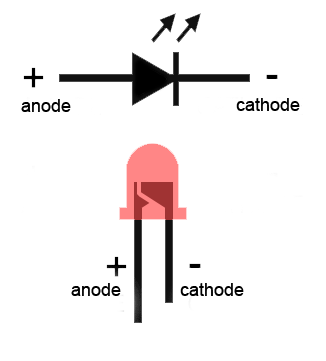# Series LED Resistor Calculator

## This series LED resistance calculator is perfect for when you have a single LED or a number of the same color of LEDs in series and need to know which resistor you should use.

(Vs)
(Vf)
If (mA)

(Ω)
(W)
(W)

### Overview

Every light emitting diode (LED) has a current that it can safely handle. Going beyond that maximum current, even briefly, will damage the LED. Thus, limiting the current through the LED with the use of a series resistor is a common and simple practice. Note that this method is not recommended for high current LEDs, which need a more reliable switching current regulator.

This calculator will help you determine the value of the resistor to add in series with the LEDs to limit the current. Just input the indicated values and press the "Calculate" button. As a bonus, it will also calculate the power consumed by each LED.

### Equation

$$R = \frac{V_{s}-V_{led}*N}{I_{led}}$$

Where:

$$V_{s}$$ =  Supply voltage

$$I_{led}$$ = LED current. The usual operating range of common 3 mm and 5 mm LEDs is 10-30 milliamps. If access to an LED's datasheet is impossible, 20 mA is a good guess.

$$V_{led}$$ = LED voltage drop. The voltage drop on a LED depends on the color it emits. Here is a neat table of each color and their corresponding voltage drop:

$$N$$ = number of LEDs in series

 Color Voltage Drop (V) red 2 green 2.1 blue 3.6 white 3.6 yellow 2.1 orange 2.2 amber 2.1 infrared 1.7 other 2

###An LED has a positive (anode) lead and a negative (cathode) lead. The schematic symbol of the LED is similar to the diode (as shown above) except for two arrows pointing outwards. The anode (+) is marked with a triangle and the cathode (-) is marked with a line.

The longer lead of an LED is almost always the positive (anode) lead, while the shorter one is the negative (cathode). Also, if you look inside the LED, the smaller of the metal pieces is connected to the anode while the bigger one is connected to the cathode (see diagram above).

Textbook - Simple Series Circuits

Textbook - Building Simple Resistor Circuits

Textbook - Light Emitting Diodes• R
ROBCHANGEDcalcfan April 08, 2016

The presentation is excellent.  It is complete with the terminal identifications and typical LED colors.  As a technical writer myself I especially appreciate a complete and practical presentation.

Many thanks,

X < > Y,
calcfan

Like.
• A
arike123 April 16, 2016

there is a very important parameter that this calculation requires to provide = the power disipation of the resistor.
when choosing the resistor to put in series with the led , important to know what size of the resistor to choose: 0125W , 0.25W . 0.5W , 1W , ..... it is more important then the value itself. I can choose a large spectrum of values of the resistance and it will work but if i will choose the wrong size it will burn.!

Like.
• K
Kris Parker March 27, 2022

This is a great tool to use.

Like.